# Use Binary Number System to Guess A Number

We all know numbers and use them in our everyday life. There are different number systems in mathematics. The decimal system is the one most commonly used. In this article, we will use the binary system to create a fun classroom activity. The binary number system uses only 0 and 1. We can convert binary numbers to decimal numbers and vice versa. As the decimal number system has ones, tens, hundreds, and thousands, e.t.c, the binary number system has ones, twos, fours, eights, and so on.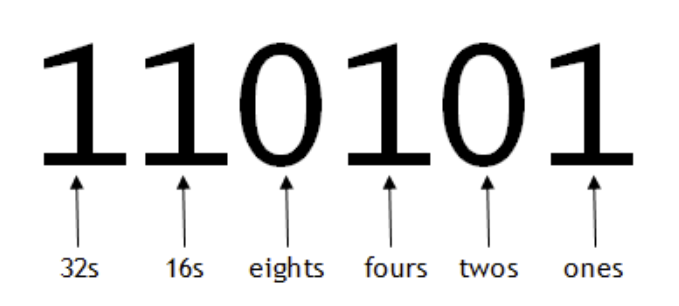Here is an example of how we can convert a binary to decimal: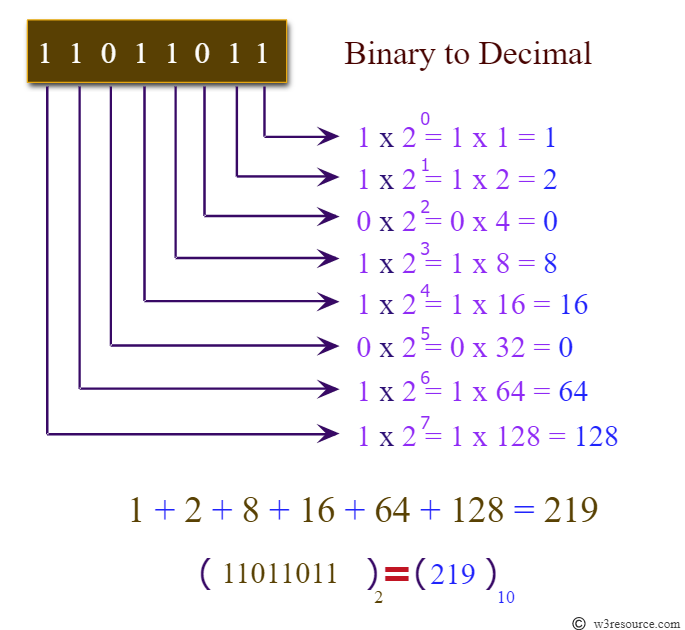Please watch this 2-minute youtube video to see how easily we can convert binary numbers to decimal numbers.   We can use this trick to create magic cards to guess the number. We will need six cards to guess any number between 1 and 63. For larger numbers, we will need more cards. Card 1: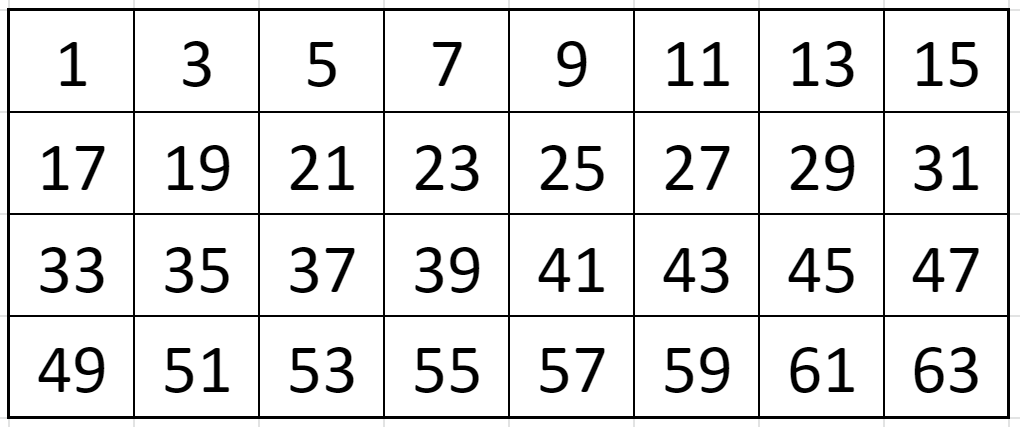Card 2: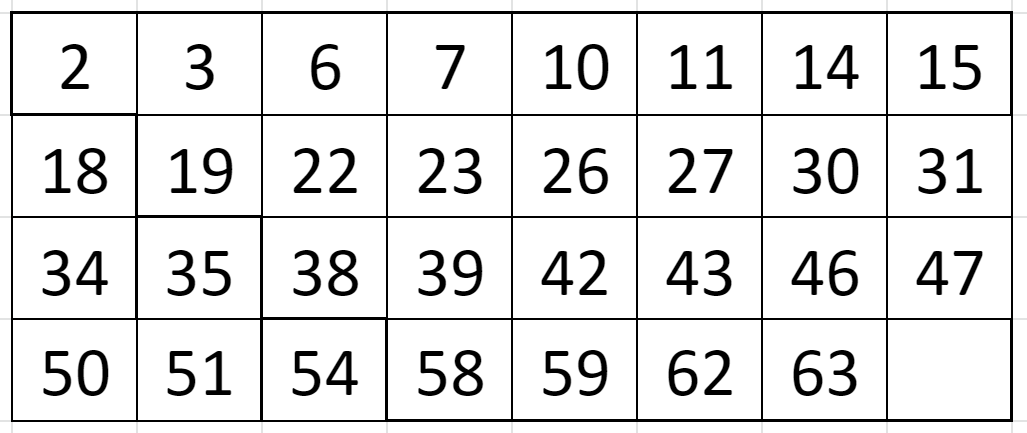Card 3: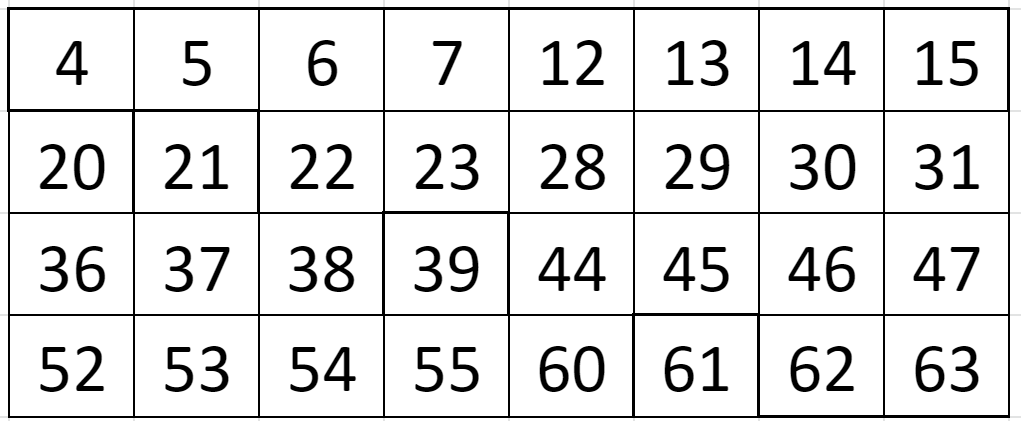Card 4:Card 5: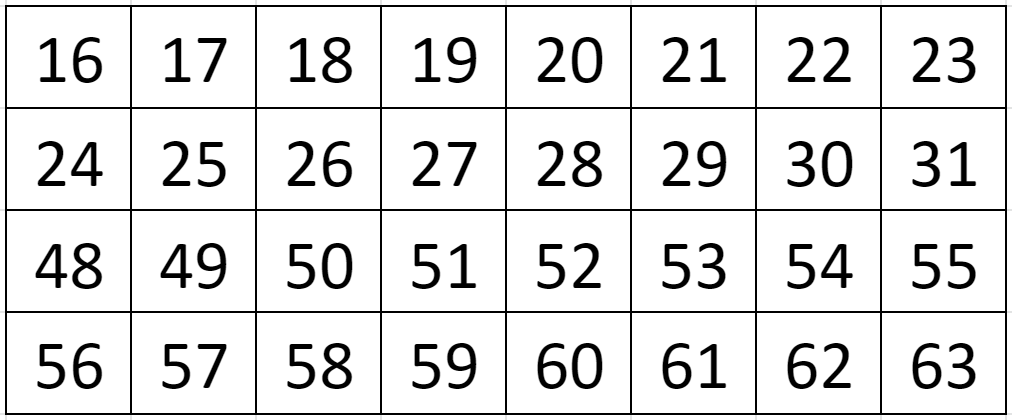Card 6: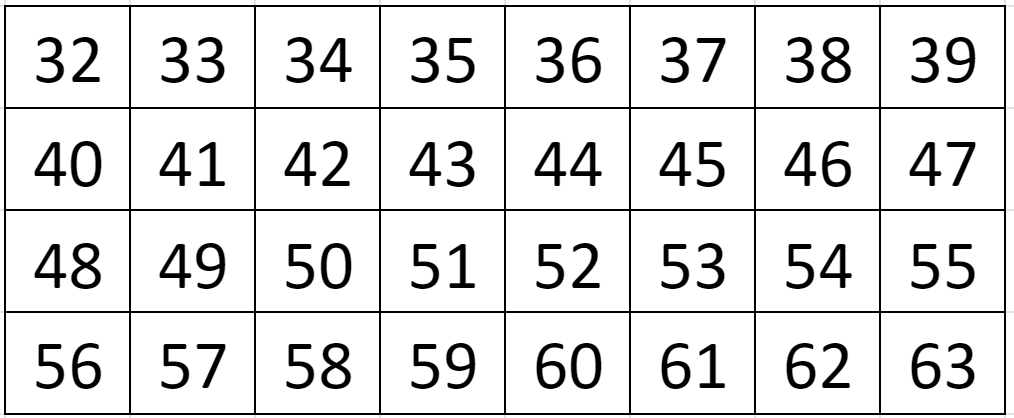Let’s try it with a number, for example, 17. We will ask the question “is your number on the card” for each card in order and see if 17 is on the card or not. Card 1: Yes – add the first number on the card.  +1 Card 2: No – skip Card 3: No – skip Card 4: No – skip Card 5: Yes – add the first number on the card.  +16 Card 6: No – skip From right to left we will have the binary number 010001 when we convert yes to 1 and no to zero. 010001 is 1 + 16 = 17. Here is another example, 35: Card 1: Yes – add the first number on the card. +1 Card 2: Yes – add the first number on the card.  +2 Card 3: No – skip Card 4: No – skip Card 5: No – skip Card 6: Yes – add the first number on the card. +32 From right to left we will have the binary number 100011 which is 1 + 2 + 32 = 35. The trick is that cards have the powers of 2 as their first number. Ask the question “is your number on the card” for each card. You add the first number on the card if it is a yes and skip if it is a no. It is that simple. Now, it is your turn to have a fun math activity with your students.